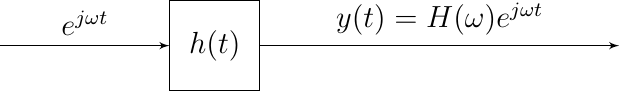# 2.4. Eigenfunctions¶

A remarkable fact of linear systems is that the complex exponentials are eigenfunctions of a linear system. I.e. if we take a complex exponential $$x(t)=\exp(j\omega t)$$ as input, the output is a complex exponential, with the same frequency as the input but multiplied with a complex constant (dependent on the frequency).Fig. 2.12 Complex Exponentials are the Eigenfunctions of a CT LTI Linear System

Consider the system with impulse response $$h$$ then the output is given by:

$y(t) = \int_{-\infty}^{\infty} e^{j\omega(t-u)} h(u) du$

We can simplify this as

$y(t) = e^{j \omega t} \int_{-\infty}^{\infty} e^{-j\omega u} h(u) du$

Observe that the integral only depends on $$\omega$$ and we denote it as $$H(\omega)$$, then:

$y(t) = e^{j \omega t} H(\omega)$

i.e. in case the input of a linear system is a sinusoidal signal (complex exponential) the output is the exponential function (sinusoidal function with the same frequency) multiplied with a complex factor $$H(\omega)$$ that is completely characterized by the linear system (its impulse response).

The function $$H$$

(2.2)$H(\omega) = \int_{-\infty}^{\infty} e^{-j\omega u} h(u) du$

is called the Fourier transform of $$h$$. The Fourier transform will play a major role in this lecture series.

Let’s redo this analysis for a real valued function: $$x(t)=\cos(\w t)$$. We know that

$x(t) = \cos(\w t) = \half (e^{j\w t} + e^{-j\w t})$

Because we are considering a linear system we have:

$\begin{split}y(t) &= (\op Lx)(t) = \half( \op L(e^{j\w t}) + \op L(e^{-j\w t}) )\\ &= \half\left( H(\w)e^{j\w t} + H(-\w) e^{-j\w t} \right)\end{split}$

Note that from the definition of the Fourier transform (Eq. (2.2))we can see that if $$h$$ is real valued function (which in practice it always is) that $$H(-\w) = H^\star(\w)$$. Writing $$H(\w)$$ in polar notation:

$\begin{split}H(\w) &= |H(\w)| e^{j\angle H(\w)}\\ H(-\w) &= H^\star(\w) = |H(\w)| e^{-j\angle H(\w)}\end{split}$

So

$\begin{split}y(t) &= \half |H(\w)| \left( e^{j(\w t+\angle H(\w)} + e^{-j(\w t +\angle H(\w)} \right)\\ &= |H(\w)| \cos( \w t + \angle H(\w))\end{split}$

So for a linear system with impulse response $$h$$ we have

$x(t) = e^{j\w t} \quad\xrightarrow{\quad L\quad}\quad y(t) = H(\w) e^{j\w t}$

and

$x(t) = \cos(\w t) \quad\xrightarrow{\quad L\quad}\quad y(t) = |H(w)| \cos( \w t + \angle H(\w))$

Evidently the use of complex exponential functions allows for a much more convenient (and short) notation. Complex numbers are really indispensible in signal processing theory.